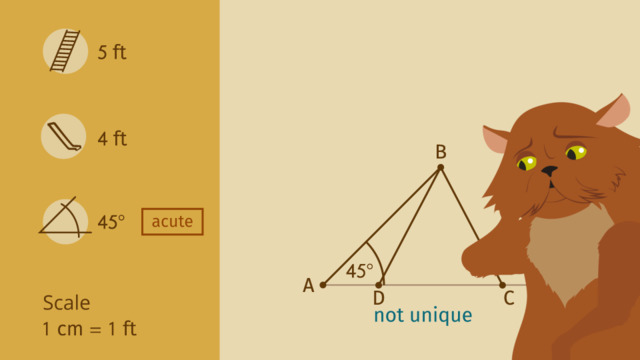# Determining a Unique Triangle Given Two Sides and a Non-included Angle.Rating

Be the first to give a rating!

The authorsChris S.

## Basics on the topicDetermining a Unique Triangle Given Two Sides and a Non-included Angle.

After this lesson you will understand the conditions required for determining a unique triangle, given two sides and a non-included angle.

The lesson begins with a review of unique triangles and the different measurements that make up all triangles. It continues by showing three examples of constructing triangles given the measurements of two sides and a non-included angle (obtuse, acute, and right).

Learn about unique triangles by helping the Pharaoh (and his cat) build a waterslide!

This video includes key concepts, notation, and vocabulary such as: unique triangle (a triangle that can only be drawn one way); acute angle (an angle that is less than 90 degrees); right angle (an angle that measures 90 degrees); and obtuse angle (an angle that is greater than 90 degrees).

Before watching this video, you should already be familiar with the concept of unique triangles, and different kinds of angles.

After watching this video, you will be prepared to explore two-dimensional figures that result from slicing three-dimensional figures.

Common Core Standard(s) in focus: 7.G.A.2 A video intended for math students in the 7th grades Recommended for students who are 12-13 years old